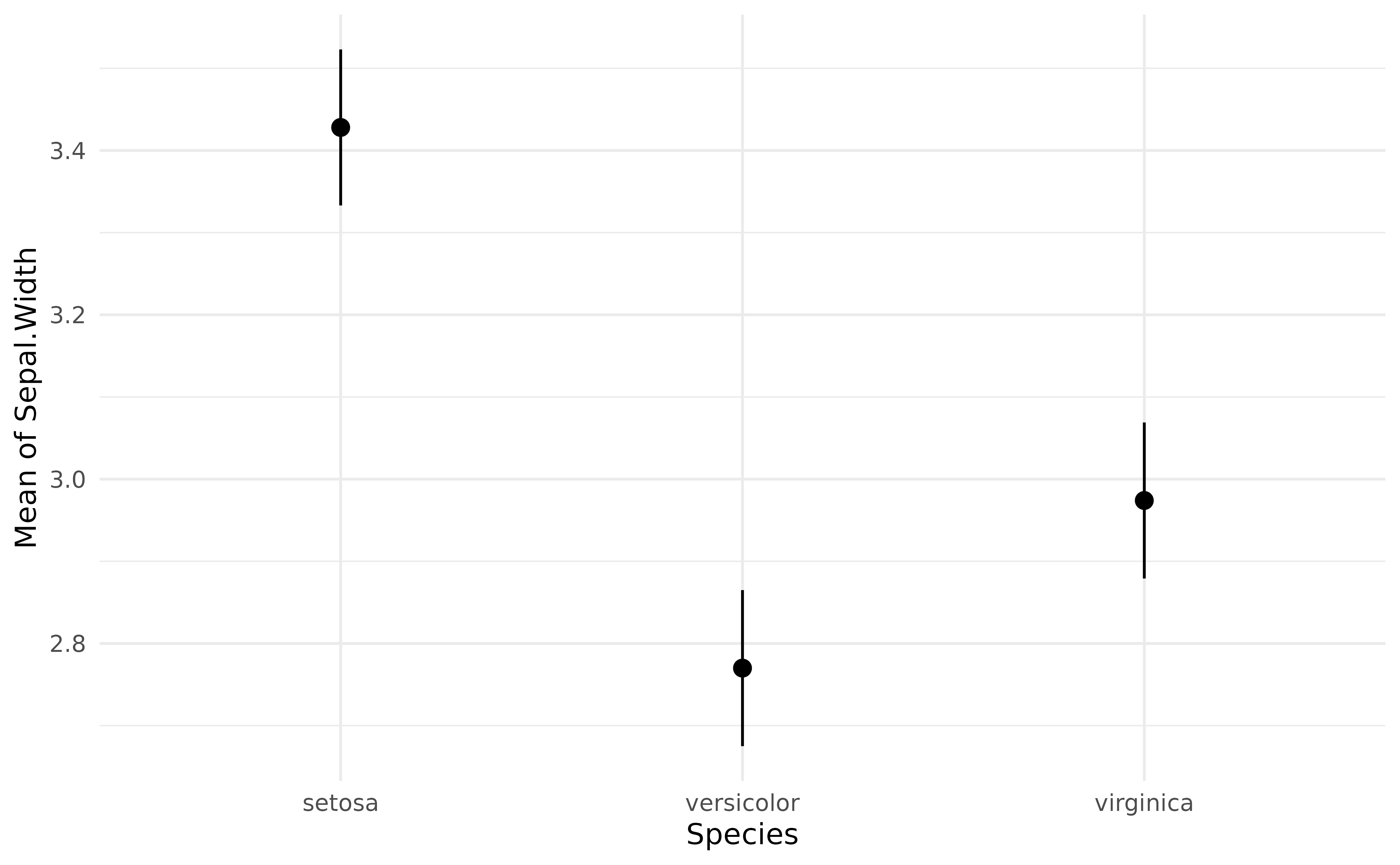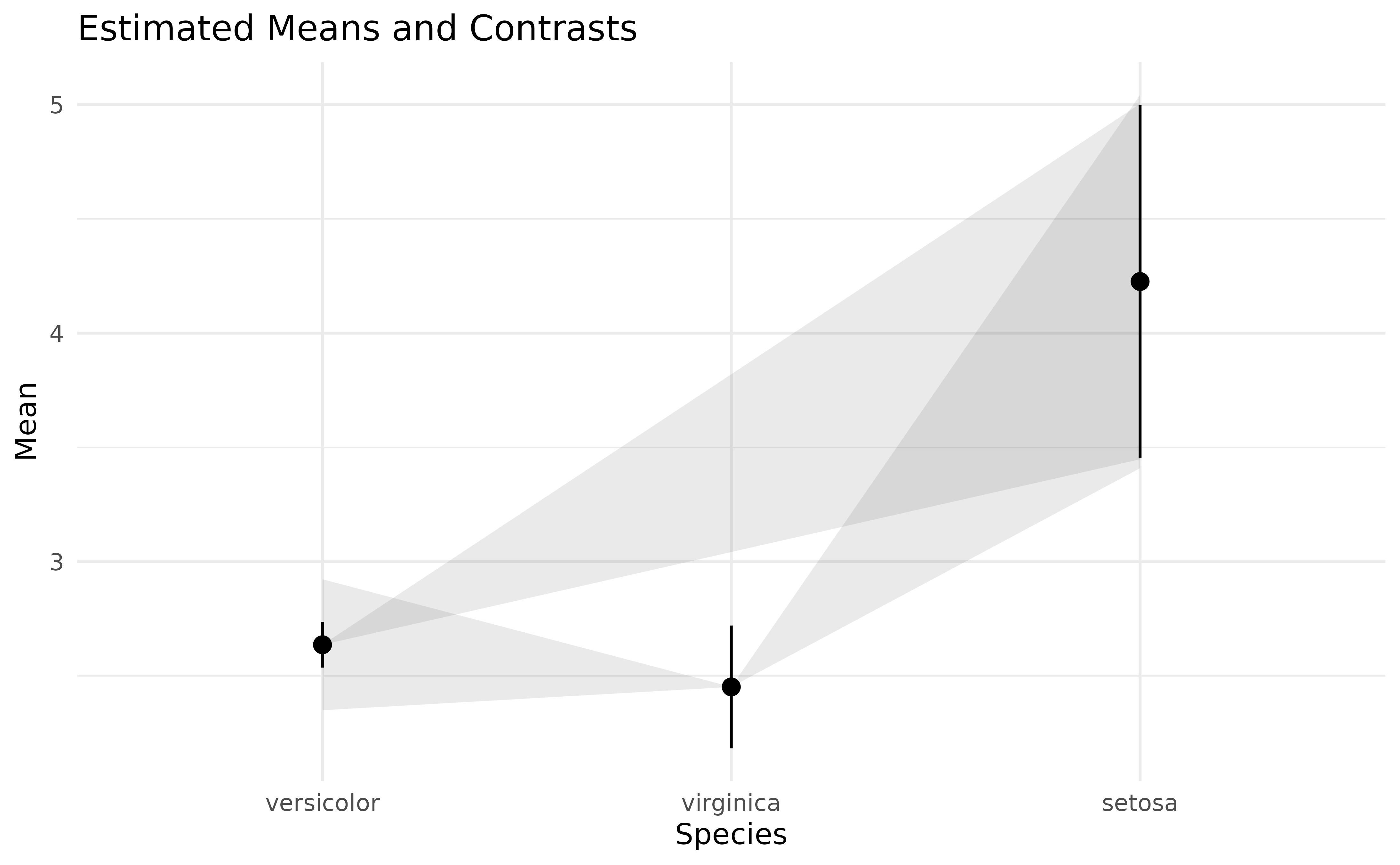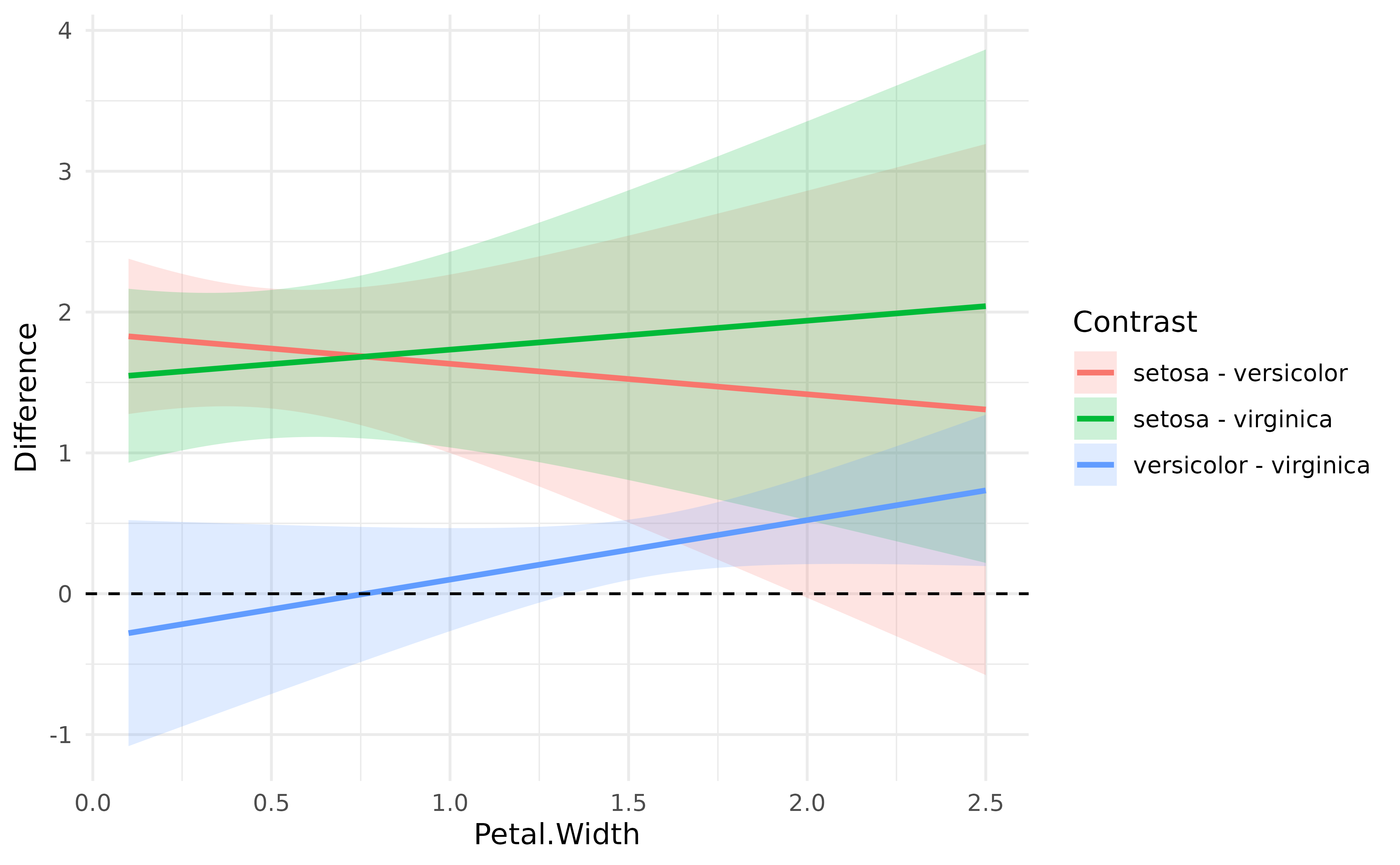## Testing pairwise differences

In the previous tutorial, we computed marginal means at the 3 different Species levels from the iris dataset. However, one might also want to statistically test the differences between each levels, which can be achieved through contrast analysis. Although the procedure is much more powerful, its aim is analogous to the post hoc analysis (pretty much consisting of pairwise t-tests), which are heavily utilized in behavioral sciences as a way to follow up on hypotheses about global differences tested by ANOVAs with more specific hypotheses about pairwise differences.

Let’s carry out contrast analysis on the simple model from the previous tutorial:

library(ggplot2)
library(see)
library(modelbased)

model <- lm(Sepal.Width ~ Species, data = iris)
means <- estimate_means(model)

ggplot(means, aes(x = Species, y = Mean)) +
geom_line(aes(group = 1)) +
geom_pointrange(aes(color = Species, ymin = CI_low, ymax = CI_high)) +
theme_modern()Contrast analysis can be achieved through the estimate_contrasts function:

estimate_contrasts(model)
> Marginal Contrasts Analysis
>
> Level1     |     Level2 | Difference |         95% CI |   SE | t(147) |      p
> ------------------------------------------------------------------------------
> setosa     | versicolor |       0.66 | [ 0.49,  0.82] | 0.07 |   9.69 | < .001
> setosa     |  virginica |       0.45 | [ 0.29,  0.62] | 0.07 |   6.68 | < .001
> versicolor |  virginica |      -0.20 | [-0.37, -0.04] | 0.07 |  -3.00 | 0.003
>
> Marginal contrasts estimated at Species
> p-value adjustment method: Holm (1979)

We can conclude that all pairwise differences are statistically significant.

## Complex model

Again, as contrast analysis is based on marginal means, it can be applied to more complex models:

model <- lm(Sepal.Width ~ Species * Petal.Width, data = iris)
contrasts <- estimate_contrasts(model)
contrasts
> Marginal Contrasts Analysis
>
> Level1     |     Level2 | Difference |        95% CI |   SE | t(144) |      p
> -----------------------------------------------------------------------------
> setosa     | versicolor |       1.59 | [ 0.64, 2.54] | 0.39 |   4.04 | < .001
> setosa     |  virginica |       1.77 | [ 0.77, 2.78] | 0.41 |   4.29 | < .001
> versicolor |  virginica |       0.18 | [-0.17, 0.54] | 0.15 |   1.27 | 0.205
>
> Marginal contrasts estimated at Species
> p-value adjustment method: Holm (1979)

For instance, if we add Petal.Width in the model, we can see that the difference between versicolor and virginica becomes not significant (and even changes sign).

Note that we can plot simple contrast analysis through lighthouse plots with the help of the see package:

library(see)

plot(contrasts, estimate_means(model)) +
theme_modern()These represent the estimated means and their CI range (in black), while the grey areas show the CI range of the difference (as compared to the point estimate).

## Changes in difference

Interestingly, we can also see how these differences are modulated by another continuous variable. Based on the model above (including the interaction with Petal.Width), we will compute the contrasts at 100 equally-spaced points of Petal.Width, that we will then visualise.

contrasts <- estimate_contrasts(model, at = "Petal.Width", length = 100)

# Create a variable with the two levels concatenated
contrasts$Contrast <- paste(contrasts$Level1, "-", contrasts\$Level2)

# Visualise the changes in the differences
ggplot(contrasts, aes(x = Petal.Width, y = Difference)) +
geom_ribbon(aes(fill = Contrast, ymin = CI_low, ymax = CI_high), alpha = 0.2) +
geom_line(aes(colour = Contrast), size = 1) +
geom_hline(yintercept = 0, linetype = "dashed") +
theme_modern() +
ylab("Difference")As we can see, the difference between versicolor and virginica increases as Petal.Width increases.

## Conclusion

Contrast analysis can be a powerful tool to interpret and understand statistical models.